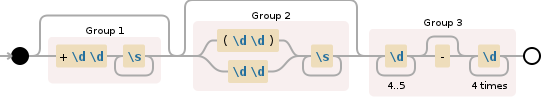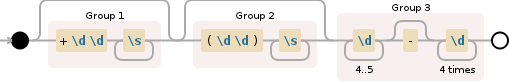# Phone number Regex not working with swift [duplicate]

I'm developing an app which works with phone numbers, but I'm having a problem using regex to find the phonenumber in a string.

My app looks through the contact's phone number which can be:

(with dash "-")
XXXX-XXXX
XXXXX-XXXXX
(YY) XXXX-XXXX
(YY) XXXXX-XXXX
+ZZ (YY) XXXX-XXXX
+ZZ (YY) XXXXX-XXXX

(without dash)
XXXXXXXX
XXXXXXXXX
(YY) XXXXXXXX
(YY) XXXXXXXXX
+ZZ (YY) XXXXXXXX
+ZZ (YY) XXXXXXXXX

Based on all this possibilities above, I've written the following code:

``````    let range = telefone.rangeOfString("[0-9]{4,5}-[0-9]{4}", options:.RegularExpressionSearch)
let range2 = telefone.rangeOfString("[0-9]{9}\$", options:.RegularExpressionSearch)

var found: String?

if range != nil{
found = telefone.substringWithRange(range!)
}else if range2 != nil{
found = telefone.substringWithRange(range2!)
}

print(found)
``````

`range` is the regex to find phoneNumbers (with dash "-")
`range2` is the regex to fund phone numbers (without dash)

With this code I get only the phone number, without the country code or the area code.

The problem is, this code returns nil on found variable when I test with a phone number like
+ZZ (YY) XXXXX-XXXX

Can someone help me find another way to write a regex to get only the "X" values of the string containing all the contact phone number?

UPDATE:

I noticed the code above, the variable range, returns null

``````    var telefone = "+42 43 23123-2221"

let range = telefone.rangeOfString("\\d{4,5}\\-?\\d{4}", options:.RegularExpressionSearch)

print("range \(range)")   //here returns nil
``````• Is it always a 2, 2, (4 or 5), 4 pattern ? You could go simple `(?:\d{2}\D*){0,2}\d{4,5}\D*\d{4}` or for a little more assurance `(?<!\d)(?:\d{2}\D*){0,2}\d{4,5}\D*\d{4}(?!\d)` – user557597 Dec 6 '15 at 19:47
• This one will capture the parts grps 1-4. `(?<!\d)(?:(\d{2})\D*)?(?:(\d{2})\D*)?(\d{4,5})\D*(\d{4})(?!\d)` demo – user557597 Dec 6 '15 at 19:54
• based on your answer i used \d{4,5}\-?\d{4}, with is the part i need from the number. but it didn't found using .rangeOfString("\\d{4,5}\\-?\\d{4}", options:.RegularExpressionSearch). i've updated my question with the complete code. Thanks!! – marchiore Dec 6 '15 at 20:57
• Does this code work for you? – Wiktor Stribiżew Dec 9 '15 at 23:45
• I have just realized that this format was not supported by the regex. Here is a working one: `pattern = "(?:(?:\\+\\d{2}\\h*)?(?:\\(\\d{2}\\)|\\d{2}))?\\h*(\\d{4,5}-?\\d{4})"`. Note that this phone number format is not mentioned in the OP. – Wiktor Stribiżew Dec 10 '15 at 21:35

This may be not the most pleasant-looking regex but I believe it does the job.

``````(\+\d{2}\s*(\(\d{2}\))|(\(\d{2}\)))?\s*\d{4,5}\-?\d{4}
``````Edit:

If I understood you correctly, this would only match the phone number without any area code etc (Just the Xs) inside a capturing group.

``````(?:\+\d{2}\s*(?:\(\d{2}\))|(?:\(\d{2}\)))?\s*(\d{4,5}\-?\d{4})
``````• i updated my question with the new regex. but it isn't working yet, it didn't find the range into the string. – marchiore Dec 6 '15 at 21:09

If the brackets are option ie. "+42 (43) 23123-2221" or "+42 43 23123-2221":

``````(?:(\+\d\d\s+)?((?:\(\d\d\)|\d\d)\s+)?)(\d{4,5}\-?\d{4})
``````Alternatively if brackets are required:

``````(?:(\+\d\d\s+)?(\(\d\d\)\s+)?)(\d{4,5}\-?\d{4})
``````You might want to replace `\s+` with simply a single space depending on your spacing requirements.

Also note you might want to add anchors `^` to the beginning and `\$` and to the end to ensure complete match of string.

https://regex101.com/r/bR5uM3/2

http://swiftstub.com/44951173

``````var telefone = "+42 43 23123-2221"

// Use this regular expression to require "+42 (43) 23123-2221" brackets
//let phoneNumberRegEx = "(?:(\\+\\d\\d\\s+)?(\\(\\d\\d\\)\\s+)?)(\\d{4,5}\\-?\\d{4})";

// Use this regular expression to make them optional "+42 43 23123-2221" brackets
let phoneNumberRegEx = "(?:(\\+\\d\\d\\s+)?((?:\\(\\d\\d\\)|\\d\\d)\\s+)?)(\\d{4,5}\\-?\\d{4})";

let range = telefone.rangeOfString(phoneNumberRegEx,
options:.RegularExpressionSearch)

print("range \(range)")

var found = telefone.substringWithRange(range!)

print(found)

let regex = try! NSRegularExpression(pattern: phoneNumberRegEx, options: [])

let telephoneRange = NSMakeRange(0, telefone.characters.count)
let result = regex.firstMatchInString(telefone, options: NSMatchingOptions(rawValue: 0), range: telephoneRange)
let r1 = result!.rangeAtIndex(1)
let r2 = result!.rangeAtIndex(2)
let r3 = result!.rangeAtIndex(3)
if (r1.length > 0) {
let phoneCountry = (telefone as NSString).substringWithRange(r1)
print("country: \(phoneCountry)")
}
if (r2.length > 0) {
let phoneArea = (telefone as NSString).substringWithRange(r2)
print("area: \(phoneArea)")
}
if (r3.length > 0) {
let phone = (telefone as NSString).substringWithRange(r3)
print("phone: \(phone)")
}
``````

This returns the following result:

``````range Optional(Range(0..<17))
+42 43 23123-2221
country: +42
area: 43
phone: 23123-2221
``````
• i used the regex (\\d{4,5}\\-?\\d{4}) because i only want the XXXX-XXXX and XXXXX-XXXX, but it only works on the online compiler, but it doesn't work on playground. I think is a bug on the ide. Can you run this code on xcode? – marchiore Dec 11 '15 at 1:20
• I've added a swiftstub link to show you the code working and further code to show the use of capture groups and extracting parts of the telephone number – Dean Taylor Dec 11 '15 at 2:11
• thanks!! i'll check it when i get home today, and post here the result! – marchiore Dec 11 '15 at 11:18
• i think the problem is with my IDE or it's a swift bug. Look at this code running on playground it's the same you run on the online compiler – marchiore Dec 12 '15 at 0:14
• debuggex.com – Dean Taylor Dec 16 '15 at 14:39

I've found the solution!

The problem is the hyphen in telefone is U+002D HYPHEN-MINUS where the hyphen in phoneNumberRegEx is U+2011 NON-BREAKING HYPHEN. When I standardised everything on U+002D, the code worked on playground.

Here is the solution, Looks a bit longer but works fine.

([+]?1+[-]?)?+([(]?+([0-9]{3})?+[)]?)?+[-]?+[0-9]{3}+[-]?+[0-9]{4}

It works for:

5417543010
541-754-3010
(541)754-3010
(541)-754-3011
+1-541-754-3010
1-541-754-3010